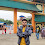# HackerRank Box It! solution in c++ programming

In this HackerRank Box It! problem in c++ programming language you need to Design a class named Box whose dimensions are integers and private to the class. The dimensions are labelled: length l, breadth b, and height h.

The default constructor of the class should initialize l, b, and h to 0.

The parameterized constructor Box(int length, int breadth, int height) should initialize Box's l,b and h to length, breadth and height.

The copy constructor Box(Box B) should set l,b and h to B's l,b and h, respectively.

Apart from the above, the class should have 4 functions:

• int getLength() - Return box's length
• int getHeight() - Return box's height
• long long CalculateVolume() - Return the volume of the box

Overload the operator < for the class Box. Box A < Box B if:

1. A.l < B.l
2. A.b < B.b and A.l==B.l
3. A.h < B.h and A.b==B.b and A.l==B.l

Overload operator << for the class Box().

If B is an object of class Box:

cout<<B should print B.l, B.b and B.h on a single line separated by spaces.

## HackerRank Box It! problem solution in c++ programming.

```#include<bits/stdc++.h>

using namespace std;
class Box
{
private:
int l,b,h;

public:
Box()
{
l = b = h = 0;
}
{
l = length;
h = height;
}
Box(const Box& B)
{
l = B.l;
b = B.b;
h = B.h;
}

int getLength()
{
return(l);
}
{
return(b);
}
int getHeight()
{
return(h);
}

long long CalculateVolume()
{
return ((long long)l*b*h);
}

friend bool operator < (Box& b1, Box& b2)
{
if((b1.l < b2.l) || (b1.l == b2.l && b1.b < b2.b) ||
(b1.l == b2.l && b1.b == b2.b && b1.h <b2.h))
return(true);
else
return(false);
}

friend ostream& operator << (ostream& s,Box& b1)
{
s << b1.l << " " << b1.b << " " << b1.h;
return s;
}
};

void check2()
{
int n;
cin>>n;
Box temp;
for(int i=0;i<n;i++)
{
int type;
cin>>type;
if(type ==1)
{
cout<<temp<<endl;
}
if(type == 2)
{
int l,b,h;
cin>>l>>b>>h;
Box NewBox(l,b,h);
temp=NewBox;
cout<<temp<<endl;
}
if(type==3)
{
int l,b,h;
cin>>l>>b>>h;
Box NewBox(l,b,h);
if(NewBox<temp)
{
cout<<"Lesser\n";
}
else
{
cout<<"Greater\n";
}
}
if(type==4)
{
cout<<temp.CalculateVolume()<<endl;
}
if(type==5)
{
Box NewBox(temp);
cout<<NewBox<<endl;
}

}
}

int main()
{
check2();
}
```

1.1.which part you need to explain < function or << function

2.2.3.